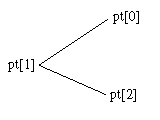﻿ L_AnnGetPoints | Function References | Raster Imaging C API Help
In This Topic ▼

# L_AnnGetPoints

#include "l_bitmap.h"

L_LTANN_API L_INT L_AnnGetPoints(hObject, pPoints)

Fills the specified array of ANNPOINT structures with the vertices of the specified annotation object.

## Parameters

### HANNOBJECT hObject

Handle to the annotation object.

### pANNPOINT pPoints

Pointer to the array that this function will fill with the vertices of the specified annotation object.

#### Returns

Value Meaning
SUCCESS The function was successful.
< 1 An error occurred. Refer to Return Codes.

## Comments

You can use the L_AnnGetPointCount function to determine the required size of the array before calling this function.

The ANNPOINT structure is like a Windows POINT structure, except that it uses double-precision floating point values.

Coordinates of an object's points are relative to its container object. The coordinates are interpreted using the container's scaling factors and offsets, which are described in Low-Level Coordinate System for Annotations.

L_AnnGetPoints works only with the following types of objects:

• ANNOBJECT_AUDIO
• ANNOBJECT_BUTTON
• ANNOBJECT_CROSSPRODUCT
• ANNOBJECT_CURVE
• ANNOBJECT_CURVECLOSED
• ANNOBJECT_ELLIPSE
• ANNOBJECT_ENCRYPT
• ANNOBJECT_FREEHAND
• ANNOBJECT_FREEHANDHOTSPOT
• ANNOBJECT_HILITE
• ANNOBJECT_HOTSPOT
• ANNOBJECT_LINE
• ANNOBJECT_NOTE
• ANNOBJECT_POINT
• ANNOBJECT_POINTER
• ANNOBJECT_POLYGON
• ANNOBJECT_POLYLINE
• ANNOBJECT_POLYRULER
• ANNOBJECT_PROTRACTOR
• ANNOBJECT_PUSHPIN
• ANNOBJECT_RECT
• ANNOBJECT_REDACT
• ANNOBJECT_RTF
• ANNOBJECT_RULER
• ANNOBJECT_STAMP
• ANNOBJECT_TEXT
• ANNOBJECT_TEXTPOINTER
• ANNOBJECT_VIDEO

For annotation objects that are defined by a rectangle, use the L_AnnGetRect function.

You can get the position of a Point object using the following:

````ANNPOINT apt; `
`L_AnnGetPoints(hPoint, &apt); ````

where hPoint is the handle to the point object.

To retrieve the points of a cross-product object, do the following:

````ANNPOINT apt; `
`L_AnnGetPoints(hPoint, apt); ````

hPoint is the handle to the cross-product object. apt and apt are the points for the primary ruler. apt is the intersection point. apt and apt are the points for the secondary ruler.

To retrieve the points of a protractor object, do the following:

````ANNPOINT apt ; `
`L_AnnGetPoints(hPoint, apt); ````

hPoint is the handle to the cross-product object. apt and apt are the endpoints for the two rulers. apt is the intersection point. This is shown below:For an ANNOBJECT_TEXTPOINTER object, `pPoints` is updated with 5 points. The first four points are coordinates of a rectangle. The last two are the endpoints of the "pointer" segment.

Note: You can not create a line of two points which they are the same.

Required DLLs and Libraries

Platforms

Win32, x64.

See Also

## Example

This example gets the points for an object, shrinks the object by 1/2
and then puts the points back onto the object.

````L_INT AnnGetPointsExample(L_INT      x, `
`                                          L_INT      y, `
`                                          HANNOBJECT hContainer)/* Container annotation object */ `
`{ `
`   L_INT nRet; `
`   HANNOBJECT  hObject;       /* Local variable for the annotation object */ `
`   POINT       PointToTest;   /* The point in the window's client area to test */ `
`   L_UINT      TestResult;    /* Result of the test */ `
`   pANNPOINT   pPoints;       /* Pointer to the points in the object */ `
`   HGLOBAL     hPoints;       /* Handle for memory management */ `
`   L_UINT      uCount;        /* Number of points in the object */ `
`   L_UINT      i; `
`   ANNRECT     rcDefine;      /* Defining rectangle for the object */ `
`   ANNRECT     rcDefineName; `
`   L_DOUBLE    cx;            /* Center point */ `
`   L_DOUBLE    cy; `
`   L_UINT      uObjectType = 0; `
`   ANNHITTESTINFO HitTestInfo; `
` `
`   /* Did we hit an object? */ `
`   /* Use incoming coordinates to specify the point to test */ `
`   PointToTest.x = x; `
`   PointToTest.y = y; `
`   /* Get the object at the specified point */ `
`   memset(&HitTestInfo, 0, sizeof(ANNHITTESTINFO)); `
`   HitTestInfo.uStructSize = sizeof(ANNHITTESTINFO); `
`   nRet = L_AnnHitTest ( hContainer, &PointToTest, &TestResult, &hObject, &HitTestInfo, sizeof(ANNHITTESTINFO)); `
`   if(nRet != SUCCESS) `
`      return nRet; `
` `
`   // Verify the hittest object is not a container `
`   L_AnnGetType(hObject, &uObjectType); `
`   if (uObjectType == ANNOBJECT_CONTAINER) `
`      return FAILURE; `
` `
`   if ( TestResult == ANNHIT_BODY ) `
`   { `
`      /* first, get the # of points in the object */ `
`      L_AnnGetPointCount ( hObject, &uCount ); `
`      /* Allocate and lock a storage for the points */ `
`      hPoints = GlobalAlloc(GPTR, sizeof(ANNPOINT) * uCount); `
`      pPoints = (pANNPOINT)GlobalLock( hPoints ); `
`      /* Now, get the points */ `
`      L_AnnGetPoints ( hObject, pPoints ); `
`      /* Get the defining rect, and find the center point */ `
`      L_AnnGetRect ( hObject, &rcDefine, &rcDefineName); `
`      cx = (rcDefine.right + rcDefine.left) / 2; `
`      cy = (rcDefine.bottom + rcDefine.top) / 2; `
`      for (i=0; i<uCount; i++) `
`      { `
`         pPoints[i].x += (cx - pPoints[i].x) / 2; `
`         pPoints[i].y += (cy - pPoints[i].y) / 2; `
`      } `
`      /* Put the new points back into the object */ `
`      L_AnnSetPoints ( hObject, pPoints, uCount ); `
`      GlobalUnlock (hPoints); `
`      GlobalFree (hPoints); `
`   } `
`   return SUCCESS; `
`} ````

Help Version 21.0.2021.4.7
Products | Support | Contact Us | Intellectual Property Notices
© 1991-2021 LEAD Technologies, Inc. All Rights Reserved.